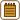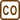## Comparison of forces

Order of magnitude comparison of forces

• gravitational force exerted by the Sun on an electron located in the Earth’s surface,
• gravitational force exerted by the proton on an electron in the hydrogen atom,
• electrical force exerted by the proton on the electron in the hydrogen atom
• #### Hint 1 – necessary values

Consider these values:

• Sun’s mass mS = 2.0·1030 kg,
• proton’s mass mp = 1.67·10−27 kg,
• electron’s mass me = 9.1·10−31 kg,
• distance from the Earth to the Sun R = 150·109 m,
• distance from the electron to the nucleus in the hydrogen atom (so-called Bohr radius of an atom)   r = 5.3·10−11 m ,
• gravitational constant κ = 6.67·10−11 N m2 kg−2,
• elementary charge e = 1.6·10−19 C,
• vacuum permittivity ε0 = 8.85·10-12 F m-1.

• #### Solution

The magnitude of the gravitational force acting between the Sun and the electron on the Earth’s surface is according to the Newton’s law equal to

$F_{GS} = \kappa {m_s m_e \over R^2} \approx 5.4 {\cdot} 10^{-33}\,.$

The magnitude of the gravitational force acting between the proton and the electron in the hydrogen atom is according to the Newton’s law equal to

$F_{gp} = \kappa {m_p m_e \over r^2} \approx 3.6 {\cdot} 10^{-47}\,.$

The magnitude of the electrical force acting between the proton and the electron in the hydrogen atom is according to the Coulomb’s law equal to

$F_{ep} = {1 \over 4 \pi \epsilon_0} {e^2 \over r^2} \approx 8.2 {\cdot} 10^{-8}\,.$

The ratio between electrical and gravitational forces between the proton and the electron in the hydrogen atom is in order of magnitude equal to

${F_{ep} \over F_{gp}} \approx 10^{39}.$

The ratio between the electrical force between the proton and the electron in the hydrogen atom and the gravitational force between the Sun and the electron on the Earth’s surface is in order of magnitude equal to

${F_{ep} \over F_{gS}} \approx 10^{25}.$

From the comparison we can see that the electrical force is much stronger than the gravitational force even when it comes to small charges against great masses. This problem illustrates why we can neglect the effect of gravitation during computation of the hydrogen atom.

• #### Komentář

The fact that gravitation compared to other interactions is weak makes it more complicated for physicist to create the quantum gravitational theory. Nowadays there are couple of final candidates for this theory such as the M-theory, which includes the superstring theory, loop quantum gravity theory, theory of supergravity and others. These theories are widely complex and were made in the way so that they give same results as general relativity in reachable conditions. However, the main problem is with testing their predictions in extreme conditions, which we are still unable to recreate.